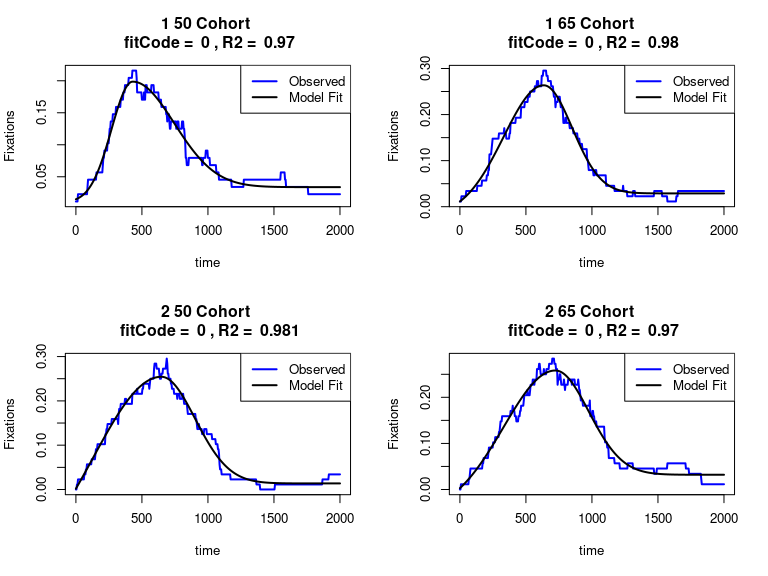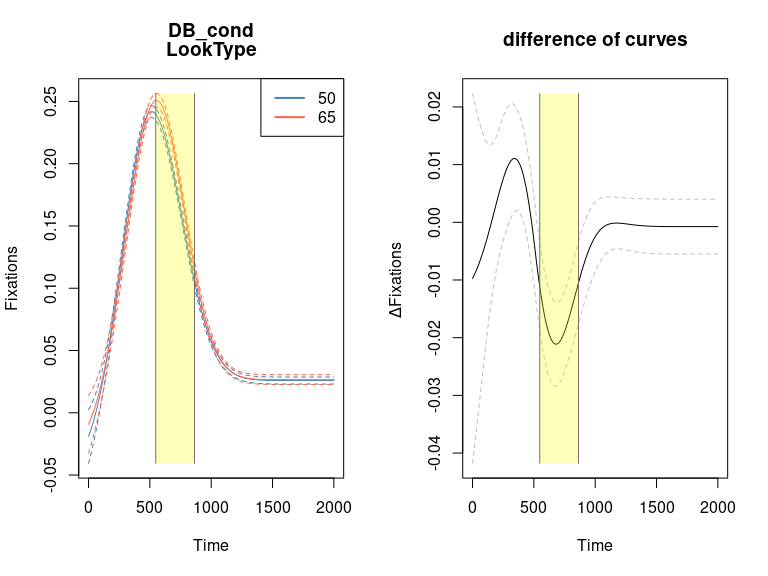bdots

library(bdots)

Overview

This vignette walks through the use of the bdots package for analyzing the bootstrapped differences of time series data. The general workflow will follow three steps:

1. Curve Fitting
During this step, we define the type of curve that will be used to fit our data along with variables to be used in the analysis
2. Curve Refitting
Often, some of the curves returned from the first step have room for improvement. This step allows the user to either quickly attempting refitting a subset of the curves from step one or to manually make adjustments themselves
3. Bootstrap
Having an adequate collection of curves, this function determines the bootstrapped difference, along with computing an adjusted alpha to account for AR1 correlation

This process is represented with three main functions, bdotsFit -> bdotsRefit -> bdotsBoot

This package is under active development. The most recent version can be installed with devtools::install_github("collinn/bdots").

Fitting Step

For our example, we are going to be using eye tracking data from normal hearing individuals and those with cochlear implants using data from the Visual Word Paradigm (VWP).

#>     Subject Time DB_cond  Fixations LookType Group
#> 502       1    0      50 0.01136364   Cohort    50
#> 503       1    4      50 0.01136364   Cohort    50
#> 504       1    8      50 0.01136364   Cohort    50
#> 505       1   12      50 0.01136364   Cohort    50
#> 506       1   16      50 0.02272727   Cohort    50
#> 507       1   20      50 0.02272727   Cohort    50

The bdotsFit function will create a curve for each unique permutation of subject/group variables. Here, we will let LookType and DB_cond be our grouping variables, though we may include as many as we wish (or only a single group assuming that it has multiple values). See ?bdotsFit for argument information.

fit <- bdotsFit(data = cohort_unrelated,
subject = "Subject",
time = "Time",
y = "Fixations",
group = c("DB_cond", "LookType"),
curveType = doubleGauss(concave = TRUE),
cores = 2)

A key thing to note here is the argument for curveType is passed as a function call with arguments that further specify the curve. Currently within the bdots package, the available curves are doubleGauss(concave = TRUE/FALSE), logistic() (no arguments), and polynomial(degree = n). While more curves will be added going forward, users can also specify their own curves, as shown here.

The bdotsFit function returns an object of class bdotsObj, which inherits from data.table. As such, this object can be manipulated and explored with standard data.table syntax. In addition to the subject and the grouping columns, we also have a fit column, containing the fit from the gnls package, a value for R2, a boolean indicating AR1 status, and a final column for fitCode. The fit code is a numeric quantity representing the quality of the fit as such:

fitCode AR1 R2
0 TRUE R2 > 0.95
1 TRUE 0.8 < R2 < 0.95
2 TRUE R2 < 0.8
3 FALSE R2 > 0.95
4 FALSE 0.8 < R2 < 0.95
5 FALSE R2 < 0.8
6 NA NA

A fitCode of 6 indicates that a fit was not able to be made.

In addition to plot and summary functions, we also have a method to return a matrix of coefficients from the model fits. Because of the data.table syntax, we can examine subsets of this object as well

#>            mu        ht     sig1     sig2        base1      base2
#> [1,] 429.7595 0.1985978 159.8869 314.6389  0.009709831 0.03376106
#> [2,] 634.9292 0.2635044 303.8080 215.3845 -0.020636088 0.02892360
#> [3,] 647.0655 0.2543769 518.9633 255.9870 -0.213087542 0.01368196
#> [4,] 723.0547 0.2582110 392.9495 252.9384 -0.054826156 0.03197291
#> [5,] 501.4822 0.2247729 500.8480 158.4180 -0.331679043 0.02522681
#> [6,] 460.7152 0.3067659 382.7321 166.0833 -0.243308563 0.03992168

#>            mu        ht     sig1     sig2        base1       base2
#> [1,] 429.7595 0.1985978 159.8869 314.6389  0.009709831 0.033761062
#> [2,] 647.0655 0.2543769 518.9633 255.9870 -0.213087542 0.013681964
#> [3,] 501.4822 0.2247729 500.8480 158.4180 -0.331679043 0.025226809
#> [4,] 521.6770 0.2483784 270.7389 209.3933 -0.038577709 0.104593300
#> [5,] 553.1884 0.2272716 207.4447 226.7181 -0.010119652 0.028663312
#> [6,] 615.9015 0.1587659 286.2063 392.5665 -0.010563154 0.007661858

The plots for this object will compare the observed data with the fitted curve. Here is an example of the first four:

plot(fit[1:4, ])Refitting Step

Depending on the curve type and the nature of the data, we might find that a collection of our fits aren’t very good, which may impact the quality of the bootstrapping step. Using the bdotsRefit function, users have the option to either quickly attempt to automatically refit specified curves or to manually review each one and offer alternative starting parameters. The fitCode argument provides a lower bound for the fit codes to attempt refitting. The default is fitCode = 1, indicating that we wish to attempt refitting all curves that did not have fitCode == 0. The object returned is the same as that returned by bdotsFit.

## Quickly auto-refit (not run)
refit <- bdotsRefit(fit, fitCode = 1L, quickRefit = TRUE)

## Manual refit (not run)
refit <- bdotsRefit(fit, fitCode = 1L)

For whatever reason, there are some data will will not submit nicely to a curve of the specfied type. One can quickly remove all observations with a fit code equal to or greater than the one provided in bdRemove

table(fit$fitCode) #> #> 0 1 2 3 4 #> 50 43 5 1 1 ## Remove all failed curve fits refit <- bdRemove(fit, fitCode = 6L) table(refit$fitCode)
#>
#>  0  1  2  3  4
#> 50 43  5  1  1

There is an additional option, removePairs which is TRUE by default. This indicates that if an observation is removed, all observations for the same subject should also be removed, regardless of fit. This ensures that all subjects have their corresponding pairs in the bootstrapping function for the use of the paired t-test. If the data are not paired, this can be set to FALSE.

Bootstrap

The final step is the bootstrapping process, performed with bdotsBoot. First, let’s examine the set of curves that we have available from the first step

1. Difference of Curves
Here, we are interested specifically in the difference between two fitted curves. For our example case here, this may be the difference between curves for DB_cond == 50 and DB_cond == 65 nested within either the Cohort or Unrelated_Cohort LookTypes (but not both).
2. Difference of Difference Curves
In this case, we are considering the difference of two difference curves similar to the one found above. For example, we may denote the difference between DB_cond 50 and 65 within the Corhort group as $$\text{diff}_{\text{Cohort}}$$ and the differences between DB_cond 50 and 65 within Unrelated_Corhort as $$\text{diff}_{\text{Unrelated_Cohort}}$$. The difference of difference function will then return an analysis of $$\text{diff}_{\text{Cohort}}$$ - $$\text{diff}_{\text{Unrelated_Cohort}}$$

We can express the type of curve that we wish to fit with a modified formula syntax. It’s helpful to read as “the difference of LHS between elements of RHS”

For the first type, we have

## Only one grouping variable in dataset, take bootstrapped difference
Outcome ~ Group1(value1, value2)

## More than one grouping variable in difference, must specify unique value
Outcome ~ Group1(value1, value2) + Group2(value3)

That is, we might read this as “difference of Outcome for value1 and value2 within Group1.”

With our working example, we would find the difference of DB_cond == 50 and DB_cond == 65 within LookType == "Cohort" with

## Must add LookType(Cohort) to specify
Fixations ~ DB_cond(50, 65) + LookType(Cohort)

For this second type of curve, we specify an “inner difference” to be the difference of groups for which we are taking the difference of. The syntax for this case uses a diffs function in the formula:

## Difference of difference. Here, outer difference is Group1, inner is Group2
diffs(Outcome, Group2(value3, value4)) ~ Group1(value1, value2)

## Same as above if three or more grouping variables
diffs(Outcome, Group2(value3, value4)) ~ Group1(value1, value2) + Group3(value5)

For the example illustrated in (2) above, the difference $$\text{diff}_{50} - \text{diff}_{65}$$ represents our inner difference, each nested within one of the values for LookType. The “outer difference” is then difference of these between LookTypes. The syntax here would be

diffs(Fixations, DB_cond(50, 65)) ~ LookType(Cohort, Unrelated_Cohort)

Here, we show a fit for each

boot1 <- bdotsBoot(formula = Fixation ~ DB_cond(50, 65) + LookType(Cohort),
bdObj = refit,
Niter = 1000,
alpha = 0.05,
cores = 2)

boot2 <- bdotsBoot(formula = diffs(Fixation, LookType(Cohort, Unrelated_Cohort)) ~ DB_cond(50, 65),
bdObj = refit,
Niter = 1000,
alpha = 0.05,
cores = 2)

For each, we can then produce a model summary, as well as a plot of difference curves

summary(boot1)
#>
#> bdotsBoot Summary
#>
#> Curve Type: doubleGauss
#> Formula: Fixations ~ (Time < mu) * (exp(-1 * (Time - mu)^2/(2 * sig1^2)) * (ht - base1) + base1) + (mu <= Time) * (exp(-1 * (Time - mu)^2/(2 * sig2^2)) * (ht - base2) + base2)
#> Time Range: (0, 2000) [501 points]
#>
#> Difference of difference: FALSE
#> Paired t-test: TRUE
#> Difference: DB_cond
#>
#> Autocorrelation Estimate: 0.99787